Coordinate systems and change of basis

Due to the short length of this course, we will not be covering the topic of least squares as previously advertised. This lecture will be about change of basis.
Two examples will illustrate the ideas:
• Magic squares
• Population movement

Definitions:
5: Coordinate system:
A coordinate system is a basis that is used to describe points in the vector space
6: Change of basis:
A change of basis is a description of how to get from a description of points in terms of one basis to a description in terms of another. Eg, (1,1) and (1,-1) form a basis of R2, call it B. Then (0,1)=(1/2)(1,1)-(1/2)(1,-1), so (0,1) is 1/2 of one vector, and -1/2 of the other, so we write this as (0,1)=(1/2,-1/2)B. This is a change of basis from the standard basis to the basis B. This can be given in terms of a matrix.

Notes on text book:
5.3: Linear independent sets, basis
We've already seen the ideas of this section. One reason a vector space is such a great thing is that all the infinitely many things in it can be described by just a few - you need some vectors that will span it, eg, (1,0) and (0,1) span the two dimensional space R2, since you can get to any other point using them. They are also linearly independent; you could take (1,0), (0,1), and (1,1), but that is more than we need. A basis is a collection of vectors that is just right; not too little, not too much. We'll also mention orthogonal basis a little, which is from section 7.1 and 7.2. Orthogonal basis come up when we find the eigen vectors of a symmetric matrix.
5.4: Coordinate systems
This is about when you choose a different basis. It's about how something is in the span of a certain set, eg, (1,1)=1(1,0)+1(0,1), but in another coordinate system, eg, that with basis (1,1), (1,-1), we get (1,1)=1(1,1)+0(1,-1).
5.7: Change of basis
Section 5.4 talks about different basis. This section gives a rule for how to change from a description of a point in terms of one basis to a description in terms of another. Eg, how to quicly work out how something is in the span of a different choice of vectors, eg, what is (1,1) in terms of (1,1) and (1,-1) rather than in terms of (1,0) and (0,1)? We will also look at reasons why you might want to do this, in particular, the population Markov chain. When we look at orthogonal systems, we'll see a really easy way to change basis, and see a use of the projection map.

Basis and coordinate systems

We've already seen how we need to find how something is in the span of the eigen vectors to find what happens in some process in the long run. In those examples, the eigen vectors are a linearly independent spanning set, and so formed a basis. When we expressed the initial vector in terms of the eigen vectors, we were making a change of coordinate system.
There are other situations were one might want to change basis, or it might not be clear what is the best basis. We'll look at some examples.

Example: Magic Squares

The problem is about finding magic squares. It could be done for very large squares, but we'll just do it for a small example.
A 2 by 2 magic square is a square like this:
 a b c d
For it to be magic we must have the sums of the rows and columns all equal, so
a+b=c+d=a+c=b+d

For example, the following is a magic square, with all the rows and columns adding up to 10.
 7 3 3 7

What are all the possible magic squares?
The set of magic squares is a vector space, since if we add two magic squares, or multiply by a constant, we still have a magic square:
 a b c d
+
 e f g h
=
 a+e b+f c+g d+h
k
 a b c d
=
 ka kb kc kd

Eg, if we have a magic square whos rows and cols add to 8, and another whos rows and cols add to 7, then the sum is a magic square whos rows and cols add to 15.
We can make this more like a normal linear algebra problem that we're used to by making squares correspond to points in R4.
The space of all 2 by 2 squares corresponds to R4, eg,
 1 2 3 4
corresponds to (1,2,3,4), and in general
 a b c d
corresponds to (a,b,c,d).
The conditions on a,b,c,d can be written as:
 a + b = c + d a + c = b + d a + b = a + c

We can write this as follows:
 a + b - c - d = 0 a - b + c - d = 0 b - c = 0

Solve by augmented matrix and row reduction:
 / 1 1 -1 -1 | 0 \ / 1 1 -1 -1 | 0 \ / 1 1 -1 -1 | 0 \ R1-R2 / 1 0 0 -1 | 0 \ | 1 -1 1 -1 | 0 | R2-R1 | 0 -2 2 0 | 0 | -(1/2)R2 | 0 1 -1 0 | 0 | | 0 1 -1 0 | 0 | \ 0 1 -1 0 | 0 / \ 0 1 -1 0 | 0 / R3+(1/2)R2 \ 0 0 0 0 | 0 / \ 0 0 0 0 | 0 /

So the solution is
a=d and b=c

We have (a,b,c,d)=(a,b,d,c)=(d,c,c,d)=d(1,0,0,1)+c(0,1,1,0), so for the problem in R4, (1,0,0,1) and (0,1,10) is a basis for the solution space of the homogeneous system of linear equations.
The following magic squares are a basis of the space of magic squares:
 1 0 0 1
,
 0 1 1 0

So any magic square is a sum of these, eg:
6
 1 0 0 1
+ 4
 0 1 1 0
=
 6 4 4 6

The above is not the only possible basis. For instance, the following is also a basis:
 1 1 1 1
,
 1 -1 -1 1

To check this is a basis, note that they are linearly independent, and that there are two of them, so they span a space of dimension two. They are both magic squares, so they span a subspace of the space of magic squares of dimension two; but the space of magic squares itself is only of dimension two, so these must span the whole space of magic squares. We could also check this by writing linear equations, and solving them. We can also write how to get to any magic square with them, eg:
5
 1 1 1 1
+
 1 -1 -1 1
=
 6 4 4 6

What we've done is made a change of basis.
We are going to talk about how to change from a description in terms of one basis to a description in terms of another basis.
Watch this space for more to come soon.

Recurrence relation: trains and Fibonnaci sequence

I learned about the following problem in Peter Taylor's class on math and poetry (highly recommended), and you can find more details about this, and lots of variations on it, in his high school math text book.
We have carriages that are length 1 or two, and these can be used to make trains.
The carriages: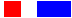Some trains: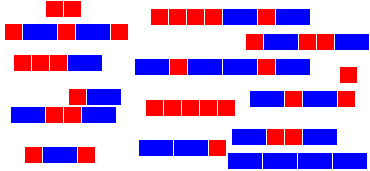The problem is, how many trains can you make of a certain length?
For example, how many trains are there of length 5?
Well, you can play around and find that the below are all the possibilities: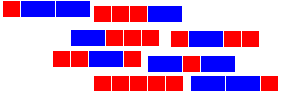To make sure you've got them all, you have to think of some way of thinking about them. Eg, you could think about trains with two carriages of length 2, or one, or no carriages of length 2: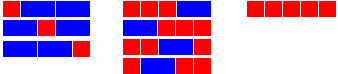Or another way is to pick out which trains start with carriages of length one, and which start with carriages of length 2: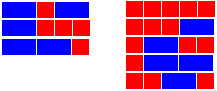This second way turns out to be very useful, since now, if we cut off the front carriage, in one case we have trains of length 4 left over, and in the other case, trains of length 3.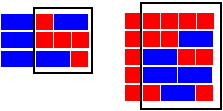So, now we can see that
Number of trains of length 5 = number of trains of length 4 + number of trains of length 3
And in general, the same kind of thing is going to work, we have a sequence of relations:
If tn is the number of trains of length n, then we have:
t2=t1+t0
t3=t2+t1
t4=t3+t2
t5=t4+t3
t6=t5+t4
t7=t6+t5
t8=t7+t6
and so on: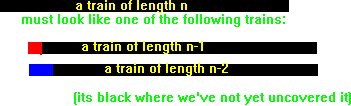tn=tn-1+tn-2

 n tn 1 2 3 4 5 6 7 8 9 10 1 2 3 5 8 13 21 34 55 89

This is a linear relation; in terms of vectors, we could write it as:
 ( tn ) = ( 1 1 ) / tn-1 \ \ tn-2 /
Well, we're applying some kind of transformation, and we want to apply it many times. But we've studied examples were you apply the transformation to something, eg, a vector (a,b) in R2, and you get out another vector in R2. In the above, you apply the transformation to two things, tn-1 and tn-2, but only get one thing out, tn. But we can make it so we get the same kind of thing out as what we put in by using two linear equations instead of just one:
tn=tn-1+tn-2
tn-1=tn-1

Now this can be written as an equation like this:
 / tn \ = / 1 1 \ / tn-1 \ \ tn-1 / \ 1 0 / \ tn-2 /

If we use some short hand:
 xn = / tn \ A = / 1 1 \ \ tn-1 / \ 1 0 /

And then the linear equations become
xn=Axn-1
eg,
x5=Ax4
x4=Ax3
x3=Ax2
x2=Ax1
x1=Ax0

So to get x5 we apply A 5 times, starting from x0. Generally we have
xn=Anx0

Actually, we do need to check that x0 makes sence, since this is (x0,x-1). Well, we can just say that there is only one way to make a train no cars long, and that is to take no carriages, and that there are no ways to get trains of length -1. So x0=(1,0).
To find xn, what we need to do is apply A n times. We've seen how to work out how to easily apply a matrix many times - find the eigen vectors and use them. More specifically:
• Find the eigen values
• Find the eigen vectors
• Find how to express the initial state in terms of the eigen vectors
• Find apply the matrix the required number of times.

Eigen values

The eigen values are (1+root(5))/2 and (1-root(5))/2. For short I'll call these t and t~ respectively. Note that t is the golden ratio.

Eigen vectors

An eigen vector for t is (t,1)
An eigen vector for t~ is (t~,1)

Initial state in terms of eigen vectors

By solving a linear system, we can find that
x0=
 / 1 \ \ 0 /
= (1/root(5))
 / t \ \ 1 /
- (1/root(5))
 / t~ \ \ 0 /

Applying An

Now we can find xn=(tn,tn-1)
xn = Anx0= An
 / 1 \ \ 0 /
= Anx0= An ( (1/root(5))
 / t \ \ 1 /
- (1/root(5))
 / t~ \ \ 0 /
)
= (1/root(5)) An
 / t \ \ 1 /
- (1/root(5)) An
 / t~ \ \ 0 /
= (1/root(5)) tn
 / t \ \ 1 /
- (1/root(5)) (t~)n
 / t~ \ \ 0 /
=
 / (1/root(5)) tn+1 - (1/root(5)) t~n+1 \ \ (1/root(5)) tn - (1/root(5)) t~n /

So, using the definition of t and t~, and substituting into the fomular, we get that the number of ways of making trains of length n is
(1/root(5))[((1+root(5))/2)n+1-((1-root(5))/2)n+1]

So, using your calculator, you can find that there are 233 ways of making trains of length 12. Can you draw them all? Will complete this soon....

Quiz

The quiz on Thursday 12th June will be similar to the following question:

Question

what is the rank of the following matrix:
 / 1 2 3 \ | 0 2 1 | \ 1 6 5 /

What is the nullity?
Find a basis for the column space
Find a basis for the null space

Solution

The rank is equal to the dimension of the column space, and also equal to the dimension of the row space, and also equal to the number of leading ones in the reduced matrix.
So we can row reduce to find the rank. Alternatively, we can just note that the third column is 2 of the first, plus half the second, and the first and second are independent, so the dimension of the column space is 2, so the rank is two.
If you don't spot the relationship between columns by inspection, you need to do some row reduction:
 / 1 2 3 \ / 1 2 3 \ / 1 2 3 \ | 0 2 1 | | 0 2 1 | | 0 2 1 | \ 1 6 5 / \ 0 4 2 / \ 0 0 0 /

 / 1 2 3 \ / 1 0 2 \ | 0 1 1/2 | | 0 1 1/2 | \ 0 0 0 / \ 0 0 0 /

In the reduced matrix, the third column is clearly 1 of the first, plus half of the second, and so the same relationship must hold in the original matrix. There are two leading ones, so the rank is 2.
The nullity is the dimension of the space of solution to the linear system with augmented matrix
 / 1 2 3 | 0 \ | 0 2 1 | 0 | \ 1 6 5 | 0 /

The rank nullity formula tells us the the nullity will be 3-2=1.
Since the first two columns are independent, and the third depends on them, the first two give a basis for the column space, so we can take for a basis:
{(1,0,1),(2,2,6)}

To find the null space, we reduce the augmented matrix to echelon form:
 / 1 0 2 | 0 \ | 0 1 1/2 | 0 | \ 0 0 0 | 0 /

So if the columns correspond to variables x,y,z, then z is the free variable, and x=-2z, y=-(1/2)z, so in terms of the free variable,
(x,y,z)=(-2z,-(1/2)z,z)=z(-2,-(1/2),1)

So every solution is a multiple of (-2,-(1/2),1), so this is a basis of the null space.

Assignment

The assignment is due in on Tuesday 17th, at my office, Jeffrey 401, before 6pm. Solutions will be posted below soon after 6pm.
Note that some exam questions may be similar to these questions. Generally exam questions will be similar to homework and quiz questions.

Note

For solutions to the assignment, see here.
Note, these are not quite completed yet. Will be by Wednesday morning.

Problem 1

(Solution)
A polynomial in x of degree 4 is something of the form:
ax4+bx3+cx2+dx+e

eg:
2x4+3x3-5x2+8x+2

The set of all polynomials of degree 4 is called P4 for short.
• (i) Show P4 is a vector space (check the first four conditions given in lecture 10
• (ii) Give a basis for P4
• (iii) Which of the following is a subspace of P4?
• (a) The set of polynomials with the coefficient of x2 =7
• (b) The set of polynomials f in P4 with f(0)=3
• (c) The set of polynomials with P4 f(1)=f(2), and f(3)=0
• (iv)
• (a) For the examples of (iii) that are not subspaces, give and example to show why not.
• (b) For the examples of (iii) that are subspaces, give a basis.

Problem 2

(Solution)
We have carriages of lengths 2 and 3.
• There are no carriages of length 1.
• There are two types of carriage of length 2, type A, and type B.
• There is one carriage of length 3, type C.

Find a formula for the number of trains of length n that can be made from these carriages.
Eg:
• for n=1, we have no ways to make a train of length 1
• For n=2, there are two ways, [AA], and [BB]
• For n=3, there is one way, [CCC]
• For n=2, there are 4 ways: [AA][BB], [AA][AA], [BB][BB], [BB][AA]

Hint:
how can you write (tn,tn-1,tn-2) in terms of (tn-1,tn-2,tn-3) if tn is the number of different types of trains of length n?
You might find it useful that the roots of
-L3+2L+1
are -1, (1-root(5))/2, (1+root(5))/2

Problem 3

(Solution)
A magic square of size 3 by 3 looks like:
 a b c d e f g h k
where we have the conditions:
a+b+c=d+e+f=g+h+k=a+d+g=b+e+h=c+f+k=a+e+k=g+e+c
• (i) Show that the set of all three by three magic squares is a vector space
• (ii) Write the conditions as 7 linear equations, and use an augmented matrix and row reduction to find a general solution
• (iii) Write a basis for the space of 3 by three magic squares